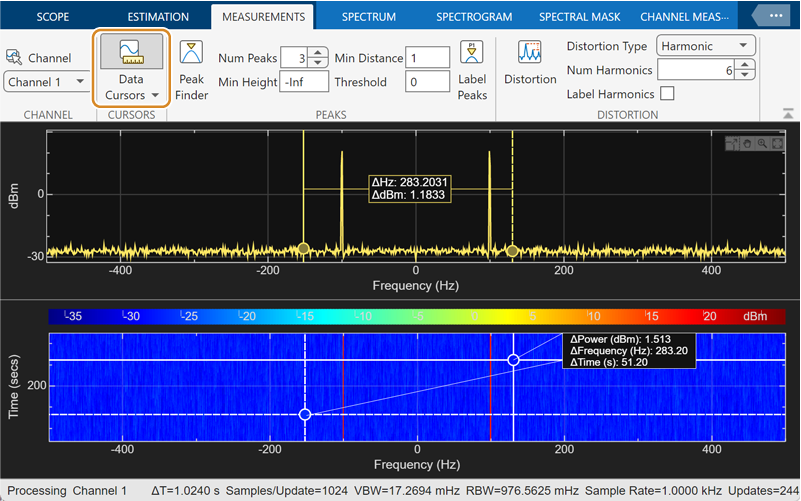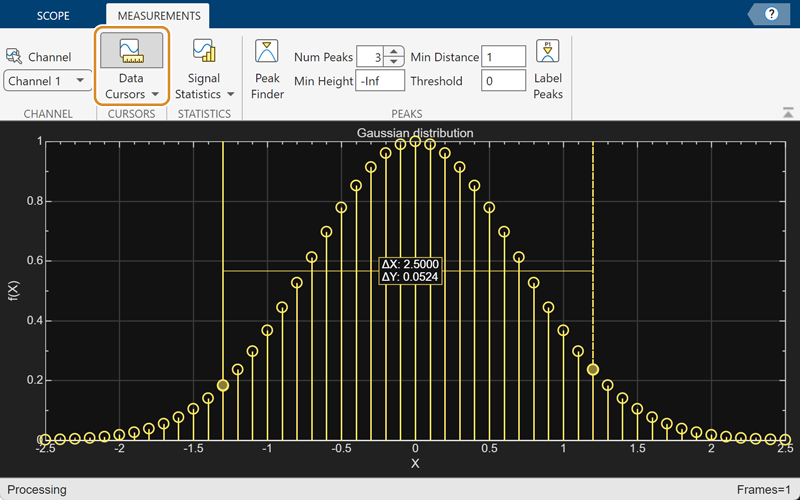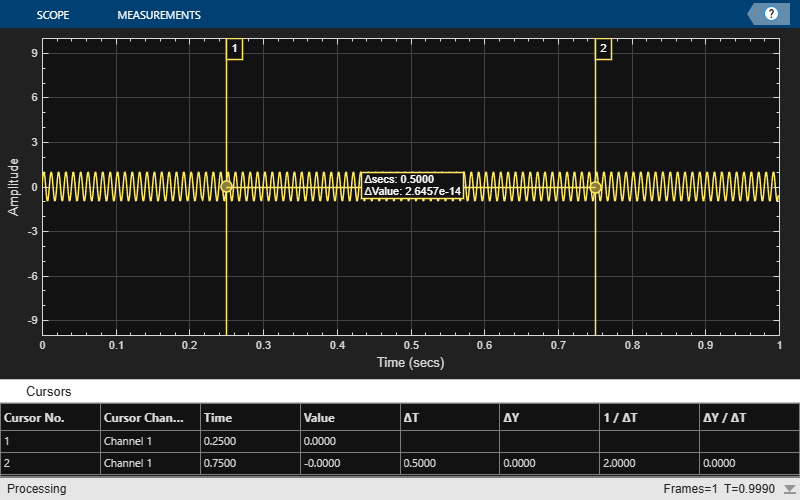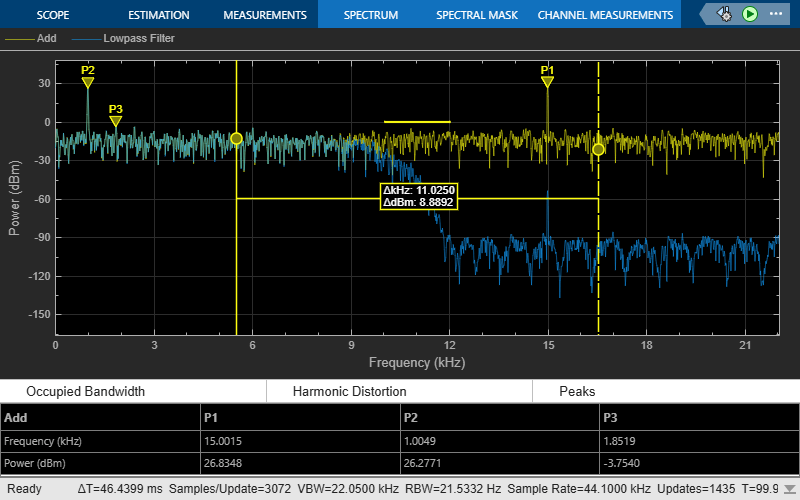# CursorMeasurementsConfiguration

Measure signal values using vertical waveform cursors that track along the signal

Since R2022a

## Description

Use the `CursorMeasurementsConfiguration` object to enable waveform cursors. You can control the cursor settings from the toolstrip of the scope or from the command line.

To display vertical cursors on each signal and to modify the cursor settings in the scope UI, click the Measurements tab and enable Data Cursors. Each cursor tracks a vertical line along the signal. The scope displays the difference between x- and y-values of the signal at the two cursors in the box between the cursors. The cursors appear only when the scope has at least one signal in its display. You can use the mouse to move the vertical cursors left and right.

Spectrum Analyzer ToolstripTime Scope ToolstripArray Plot ToolstripDynamic Filter Visualizer Toolstrip## Creation

### Syntax

``cursormeas = CursorMeasurementsConfiguration()``

### Description

example

````cursormeas = CursorMeasurementsConfiguration()` creates a cursor measurements configuration object. ```

## Properties

expand all

All properties are tunable.

x-coordinates of the cursors, specified as a two-element vector of real numbers.

#### Scope Window Use

On the Measurements tab, select Data Cursors and then click Data Cursors to specify the two elements in X location properties.

Data Types: `double`

Position cursors on the signal data points, specified as `true` or `false`.

#### Scope Window Use

On the Measurements tab, select Data Cursors and then click Data Cursors to select Snap to data.

Data Types: `logical`

Lock spacing between cursors, specified as `true` or `false`. Set this property to `true` to lock the frequency difference between the cursors.

#### Scope Window Use

On the Measurements tab, select Data Cursors and then click Data Cursors to select Lock cursor spacing.

Data Types: `logical`

Enable cursor measurements, specified as `true` or `false`. Set this property to `true` to enable cursor measurements.

#### Scope Window Use

On the Measurements tab, select Data Cursors.

Data Types: `logical`

## Examples

collapse all

Create a sine wave and view it in the Time Scope. Enable data cursors programmatically.

Initialization

Create the input sine wave using the `sin` function. Create a `timescope` MATLAB® object to display the signal. Set the `TimeSpan` property to 1 second.

```f = 100; fs = 1000; swv = sin(2.*pi.*f.*(0:1/fs:1-1/fs)).'; scope = timescope(SampleRate=fs,... TimeSpanSource="property",... TimeSpan=1);```

Data Cursors

Enable data cursors in the scope programmatically by setting the `Enabled` property of the `CursorMeasurementsConfiguration` object to `true`.

```scope.CursorMeasurements.Enabled = true; scope(swv); release(scope)```Compute and display the power spectrum of a noisy sinusoidal input signal using the `spectrumAnalyzer` MATLAB® object. Measure the peaks, cursor placements, adjacent channel power ratio, and distortion values in the spectrum by enabling these properties:

• `PeakFinder`

• `CursorMeasurements`

• `ChannelMeasurements`

• `DistortionMeasurements`

Initialization

The input sine wave has two frequencies: 1000 Hz and 5000 Hz. Create two `dsp.SineWave` System objects to generate these two frequencies. Create a `spectrumAnalyzer` object to compute and display the power spectrum.

```Fs = 44100; Sineobject1 = dsp.SineWave(SamplesPerFrame=1024,PhaseOffset=10,... SampleRate=Fs,Frequency=1000); Sineobject2 = dsp.SineWave(SamplesPerFrame=1024,... SampleRate=Fs,Frequency=5000); SA = spectrumAnalyzer(SampleRate=Fs,SpectrumType="power",... PlotAsTwoSidedSpectrum=false,ChannelNames={'Power spectrum of the input'},... YLimits=[-120 40],ShowLegend=true);```

Enable Measurements Data

To obtain the measurements, set the `Enabled` property to `true`.

```SA.CursorMeasurements.Enabled = true; SA.ChannelMeasurements.Enabled = true; SA.PeakFinder.Enabled = true; SA.DistortionMeasurements.Enabled = true;```

Use `getMeasurementsData`

Stream in the noisy sine wave input signal and estimate the power spectrum of the signal using the `spectrumAnalyzer` object. Measure the characteristics of the spectrum. Use the `getMeasurementsData` function to obtain these measurements programmatically. The `isNewDataReady` function returns `true` when there is new spectrum data. Store the measured data in the variable `data`.

```data = []; for Iter = 1:1000 Sinewave1 = Sineobject1(); Sinewave2 = Sineobject2(); Input = Sinewave1 + Sinewave2; NoisyInput = Input + 0.001*randn(1024,1); SA(NoisyInput); if SA.isNewDataReady data = [data;getMeasurementsData(SA)]; end end```The panes at the bottom of the scope window display the measurements that you have enabled. The values in these panes match the values in the last time step of the `data` variable. You can access the individual fields of `data` to obtain the various measurements programmatically.

Compare Peak Values

Use the `PeakFinder` property to obtain peak values. Verify that the peak values in the last time step of `data` match the values in the spectrum analyzer plot.

`peakvalues = data.PeakFinder(end).Value `
```peakvalues = 3×1 26.3957 22.7830 -57.9977 ```
`frequencieskHz = data.PeakFinder(end).Frequency/1000`
```frequencieskHz = 3×1 4.9957 0.9905 20.6719 ```

Compute and display the power spectrum of a noisy sinusoidal input signal using the Spectrum Analyzer block. Measure the cursor placements, adjacent channel power ratio, distortion, and peak values in the spectrum by enabling these block configuration properties:

• CursorMeasurements

• ChannelMeasurements

• DistortionMeasurements

• PeakFinder

Open and Inspect the Model

Filter a streaming noisy sinusoidal input signal using a Lowpass Filter block. The input signal consists of two sinusoidal tones: 1 kHz and 15 kHz. The noise is white Gaussian noise with a mean of 0 and a variance of 0.05. The sampling frequency is 44.1 kHz. Open the model and inspect the parameter values in the blocks.

```model = 'spectrumanalyzer_measurements.slx'; open_system(model) ```Access the configuration properties of the Spectrum Analyzer block using the `get_param` function.

```sablock = 'spectrumanalyzer_measurements/Spectrum Analyzer'; cfg = get_param(sablock,'ScopeConfiguration'); ```

Enable Measurements Data

To obtain the measurements, set the `Enabled` property to `true`.

```cfg.CursorMeasurements.Enabled = true; cfg.ChannelMeasurements.Enabled = true; cfg.DistortionMeasurements.Enabled = true; cfg.PeakFinder.Enabled = true; ```

Simulate the Model

Run the model. The Spectrum Analyzer block compares the original spectrum with the filtered spectrum.

```sim(model) ```The panes at the bottom of the spectrum analyzer window display the measurements that you have enabled.

Use `getMeasurementsData` function

Use the `getMeasurementsData` function to obtain the measurements programmatically.

```data = getMeasurementsData(cfg) ```
```data = 1x5 table SimulationTime PeakFinder CursorMeasurements ChannelMeasurements DistortionMeasurements ______________ __________ __________________ ___________________ ______________________ 9.9962 1x1 struct 1x1 struct 1x1 struct 1x1 struct ```

The values shown in the measurement panels match the values shown in `data`. You can access the individual fields of `data` to obtain the various measurements programmatically.

Compare Peak Values

As an example, compare the peak values. Verify that the peak values obtained by `data.PeakFinder` match with the values in the spectrum analyzer window.

```peakvalues = data.PeakFinder.Value frequencieskHz = data.PeakFinder.Frequency/1000 ```
```peakvalues = 26.9642 26.3047 -4.5211 frequencieskHz = 15.0015 1.0049 1.6939 ```

## Version History

Introduced in R2022a

expand all